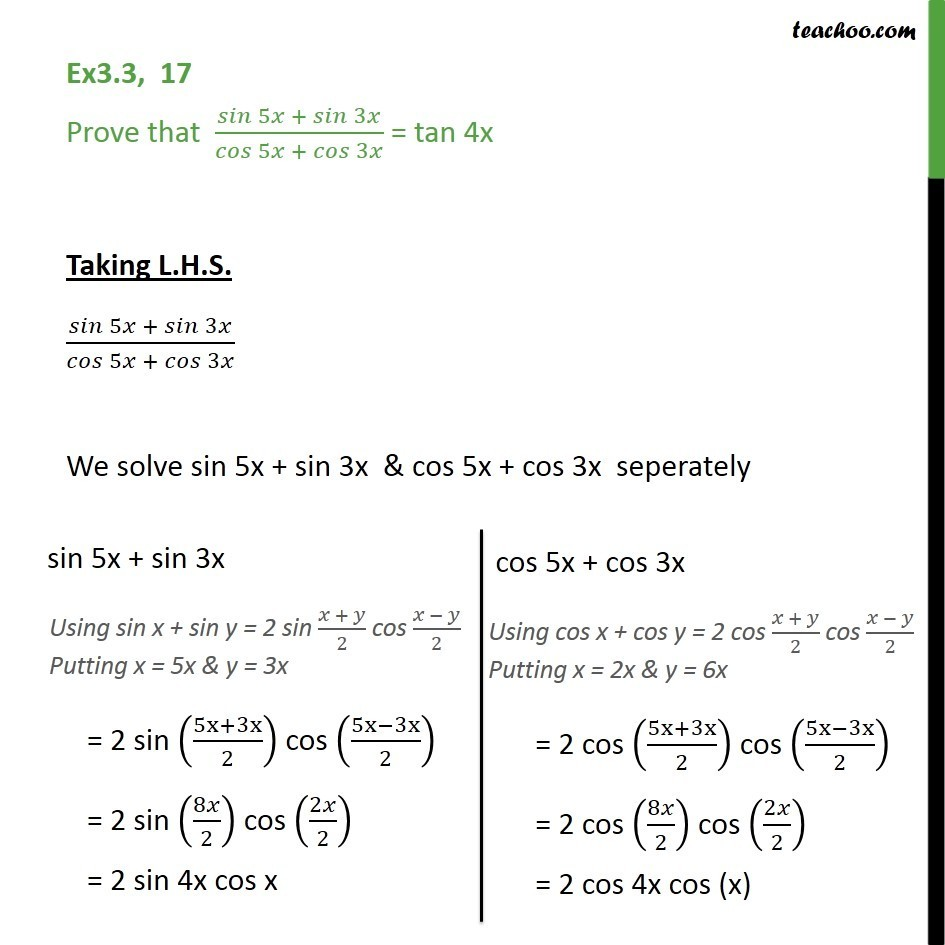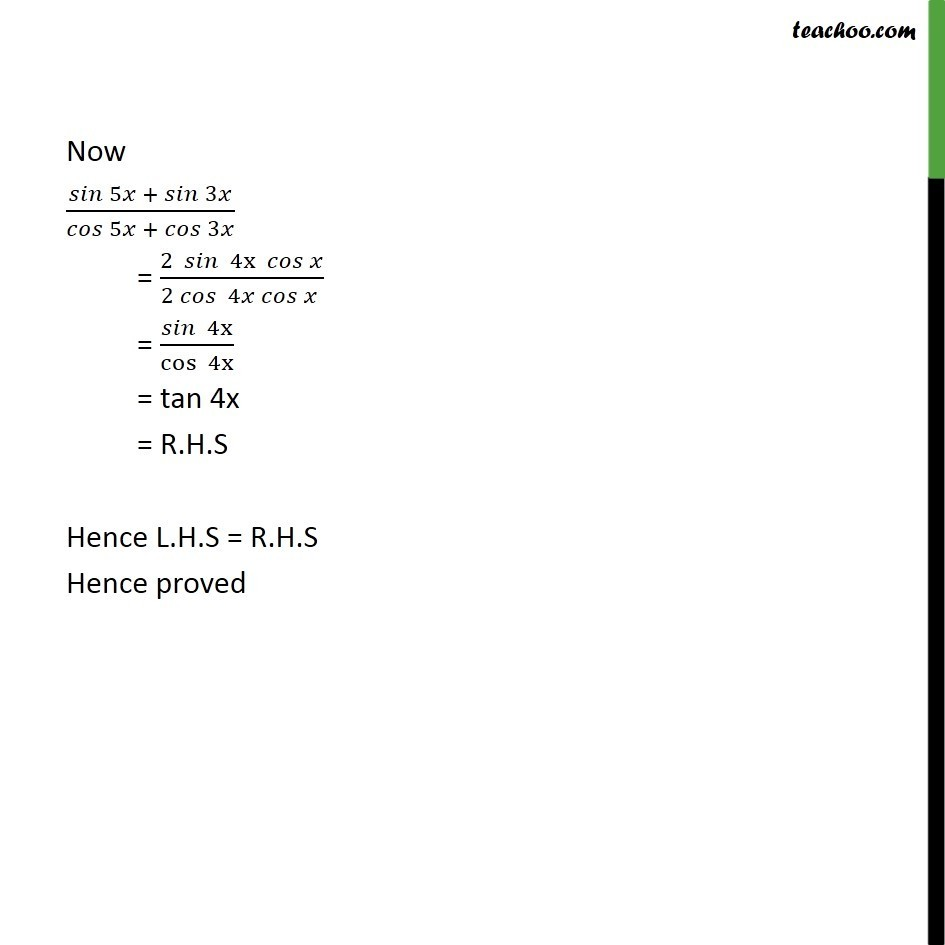Learn All Concepts of Chapter 2 Class 11 Relations and Function - FREE. Check - Trigonometry Class 11 - All Concepts1. Chapter 3 Class 11 Trigonometric Functions
2. Serial order wise
3. Ex 3.3

Transcript

Ex3.3, 17 Prove that 5 + 3 / 5 + 3 = tan 4x Taking L.H.S. 5 + 3 / 5 + 3 We solve sin 5x + sin 3x & cos 5x + cos 3x seperately Now 5 + 3 / 5 + 3 = (2 4x )/(2 4 ) = 4x /cos 4x = tan 4x = R.H.S Hence L.H.S = R.H.S Hence proved

Ex 3.3